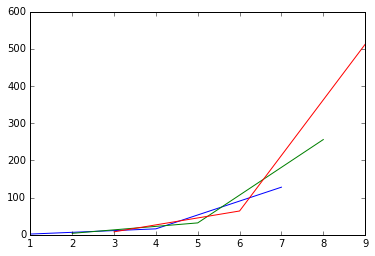Prev: What is 'matlpotlib'?       |       Next: Customizing the plot

Basic plotting with 'plot'¶

• plot is a key function of the pyplot library, used to add most major features (such as lines and markers) to the plot

How do we plot an array?¶

• Just pass the array as argument to the plot function. See the example below.
In :
import numpy as np
import matplotlib.pyplot as plt
%matplotlib inline

f = np.array([1,2,3,4,5,6,7,8,9,10])
plt.plot(f)

Out:
[<matplotlib.lines.Line2D at 0x7530748>]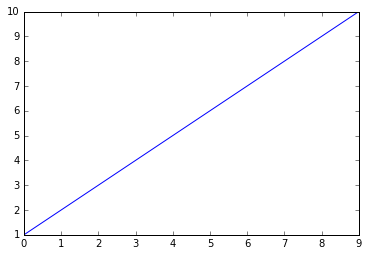• How was the above plot created?

By simply calling 'plot(f)' a graph of the array N values is plotted against the [0,N-1] values in x-axis using the default line style (blue color, continuous line)

How do I define the values in x-axis?¶

Just call plot(x, f) where x is the array with the x-axis values

In :
import numpy as np
import matplotlib.pyplot as plt
%matplotlib inline

x = np.array([1,2,3,4,5,6,7,8,9,10])/2
f = 2*x
plt.plot(x,f)

# Now the array f = 2*x is plotted against the x values [0.5, 5.0]
Out:
[<matplotlib.lines.Line2D at 0x7911e80>]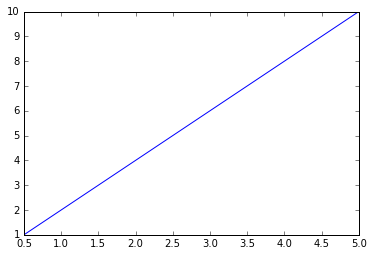How do I change the line style?¶

Add a style string as in the examples below. For example the 'g' string defines: 'green' color and asterisk ('') marks

• See more options about style strings here
In :
import numpy as np
import matplotlib.pyplot as plt
%matplotlib inline

x = np.array([i for i in range(20)])
f2 = 2*x
plt.plot(x,f2, 'g*')

f3 = 3*x
plt.plot(x,f3, 'r--')

f5 = 5*x
plt.plot(x,f5, 'm^')
Out:
[<matplotlib.lines.Line2D at 0x75c1f98>]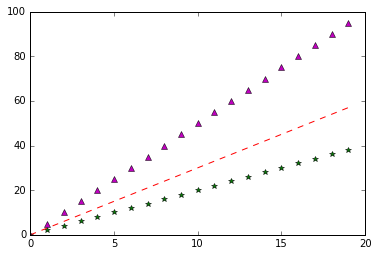• 'plot' can contain more than one groups of x, y, 'format string' values
In :
import numpy as np
import matplotlib.pyplot as plt
%matplotlib inline

x = np.linspace(0,10,30)

f1 = x
f2 = 2*x
f3 = 3*x
plt.plot(x, f1, 'k--', x, f2, 'mo', x, f3, 'g.')
Out:
[<matplotlib.lines.Line2D at 0x79e9668>,
<matplotlib.lines.Line2D at 0x79e9828>,
<matplotlib.lines.Line2D at 0x79f01d0>]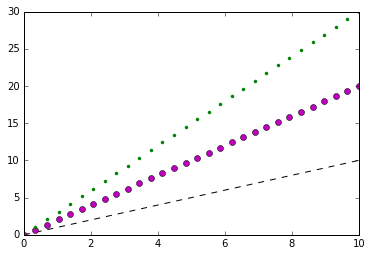• If x and/or f are 2-dimensional then the corresponding columns pairs will be plotted.

For example, the red line below is plotted based on column (x, f) pairs: (3,8), (6, 64) and (9, 512)

In :
import numpy as np
import matplotlib.pyplot as plt
%matplotlib inline

x = np.array([[1,2,3],[4,5,6],[7,8,9]]) #reshape(3,3)
f = 2**x
print(x)
print(f)
plt.plot(x, f)
[[1 2 3]
[4 5 6]
[7 8 9]]
[[  2   4   8]
[ 16  32  64]
[128 256 512]]
Out:
[<matplotlib.lines.Line2D at 0x7a56fd0>,
<matplotlib.lines.Line2D at 0x7a5d1d0>,
<matplotlib.lines.Line2D at 0x7a5d470>]